# Sampling Rate Optimization for Improving the Cascaded Integrator Comb Filter Characteristics

Sampling Rate Optimization for Improving the Cascaded Integrator Comb Filter Characteristics

Raouf AmraneYoucef Brik Samir Zeghlache Mohamed Ladjal Djamel Chicouche

LASS Laboratory, Faculty of technology, University Mohamed Boudiaf of M’sila, B.P.166, Route Ichebilia, M’sila 28000, Algeria

Corresponding Author Email:
raouf.amrane@univ-msila.dz
Page:
97-103
|
DOI:
https://doi.org/10.18280/ts.380110
4 February 2020
|
Accepted:
10 December 2020
|
Published:
28 February 2021
| Citation

OPEN ACCESS

Abstract:

The cascaded integrator comb (CIC) filters are characterized by coefficient less and reduced hardware requirement, which make them an economical finite impulse response (FIR) class in many signal processing applications. They consist of an integrator section working at the high sampling rate and a comb section working at the low sampling rate. However, they don’t have well defined frequency response. To remedy this problem, several structures have been proposed but the performance is still unsatisfactory. Thence, this paper deals with the improvement of the CIC filter characteristics by optimizing its sampling rate. This solution increases the performance characteristics of CIC filters by improving the stopband attenuation and ripple as well as the passband droop. Also, this paper presents a comparison of the proposed method with some other existing structures such as the conventional CIC, the sharpened CIC, and the modified sharpened CIC filters, which has proven the effectiveness of the proposed method.

Keywords:

CIC filter, FIR, frequency response, optimization, sampling rate, filter sharpening

1. Introduction

The filtering operation, in signal processing domain, plays a very important role in the enhancement of the signal quality. This process can be realized by removing some undesirable components or some frequency characteristics from signals (Figure 1). Nowadays, the implication of filters become indispensable for several electronics fields such as in radio, audio, telecommunication, television, radar, information transmission, ...etc. Usually, the filters can be classified as analog or digital [1-4]. In digital signal processing (DSP), there are two kinds of digital filters, the infinite impulse response (IIR) filters and the finite impulse response (FIR) filters.

The Cascaded integrator comb filter (CIC) filters, which were initially invented by Hogenauer , are a part of FIR filters that mainly used in low-cost implementation of decimation and interpolation. Besides, these filers don't require multipliers and lot of memory space, which make them an economic choice in various applications such as signal analysis, digital communication, compression, denoising, ...etc. CIC filters consist of two connected blocks in cascade, the first block is an integrator component that works at a high sampling rate and the second one is a comb component that works at a low sampling rate.

Several works have studied the CIC filters on different applications. A performance evaluation of CIC filters combined with compensation techniques have been proposed to improve the passband response of filters . This combination makes the CIC decimation component followed by the FIR decimation filter. In order to build a structure that can operate at a lower sampling rate while achieving better performances, a double sharpened CIC decimation filter has been presented . This proposed filter consists of three cascading stages as follows: the first stage is the comb decimation filter that handles at the input sampling rate. The second and the third stages are sharpened comb filters operating in low sampling rate, gradually. This scheme can produce the narrow passband droop in the sharpened second stage and then compensate it with the help of third stage.

Furthermore, the maximally flat (MF) error minimization method has been used for addressing the problem of passband droop in the compensated CIC filters . The MF method was applied in order to obtain the coefficients of the second and the fourth order compensation filters. The cascading of these two filters generated a sixth order CIC compensation filter that reduced considerably the passband drop of CIC filters. The basic structure of CIC filter has been discussed with the illustration of its important involved parameters . The authors tried to find some problems associated with the filter characteristics and emphasized a solution for improving its performance.

## 1.jpgFigure 1. Frequency magnitude response of filter

Through this study, we found that the CIC filter performances suffer from two major limitations namely the higher passband error and the lower stopband attenuation, which are undesirable in many applications . In order to remedy these problems, some improvements can be realized at the passband, stopband, or both. These enhancements improve significantly the passband and stopband attenuation.

In this work, we propose a new method based on the optimization of the CIC filter parameters to improve both the ripple and the attenuation of its stopband. This solution will examine the effect of sampling rate on the CIC filter gain response and find the optimal sampling rate value that improve efficiently the CIC filter gain response.

The remain of this paper is organized as follows: Section 2 describes the main principal of the CIC filters and their characteristics. In section 3, we explain in detail the proposed method for optimizing the CIC filter parameters. Section 4 discusses the results of the proposed method combined with some interesting examples. In order to give an idea on where our proposed method ranks performance-wise, we compare with several well-known CIC filters in section 5. Finally, the conclusions drawn from this work are in Section 6.

2. CIC Filter Review

The cascaded integrator comb (CIC) filter, which was initially proposed by Hogenauer in 1981 , is a new class of economical digital Finite Impulse Response (FIR) filters for the reason that it uses only the delays and the summation units. Usually, this filter is used in multi-modulated digital signal processing as well as in interpolation and decimation. Unlike conventional FIR filters, CIC filters have two sections called integrator and comb sections, which perform simultaneously the digital low pass filtering and decimation operations [7, 8].

CIC filters can realize the decimation by decreasing in the sampling rate and the interpolation by increasing in the sampling rate without using multipliers. A CIC filter consists of an equal number of ideal filter integrator and comb stages. Its frequency response can be adjusted by selecting the optimal number of cascade integrator and comb filter pairs . Its highly symmetric structure allows an efficient hardware implementation . Figure 2 shows the main structure of the CIC filter.

## 2.jpg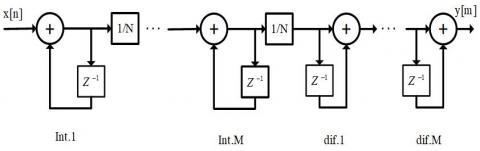Figure 2. Structure of CIC filter with multistage

In this structure, $x[n]$ designs the input signal and $y[m]$ the output signal. The transfer function of the CIC filter in z-domain is given by the following equation:

$H(\mathrm{Z}, \mathrm{N}, \mathrm{M})=\left(\frac{1}{\mathrm{~N}} \frac{1-\mathrm{Z}^{-\mathrm{N}}}{1-\mathrm{Z}^{-1}}\right)^{\mathrm{M}}$  (1)

In Eq. (1), the numerator $1-\mathrm{Z}^{-\mathrm{N}}$ represents the transfer function of comb and the denominator $1 /\left(1-\mathrm{Z}^{-1}\right)$ indicates the transfer function of integrator, where M and N denote the number of CIC stages and the decimation factor, respectively. The amplitude response of the CIC filter of the Mth order is given by:

$\left|\mathrm{H}_{\mathrm{CIC}}(\Omega, \mathrm{N}, \mathrm{M})\right|=\left|(1 / N) \frac{\sin (\mathrm{N} \Omega / 2)}{\sin \left(\frac{\Omega}{2}\right)}\right|^{\mathrm{M}}$  (2)

where, $\Omega$ represents the normalized frequency.

a)

## 3a.jpg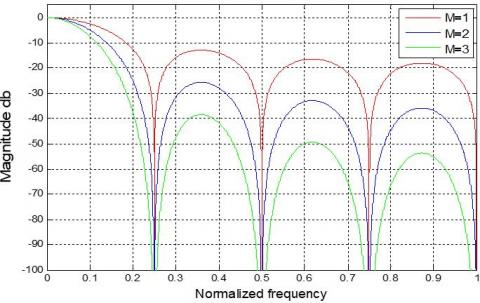b)

## 3b.jpgFigure 3. CIC filter gain responses with N=8 and M=1, 2, and 3: a) the whole magnitude response, b) the passband zoom

The illustrations depicted in Figure 3 represent the frequency response of CIC filter for N=8 with three different value of M. We note that when increasing the number of stages M, the passband droop decreases and the stopband attenuation augmented constantly.

## 4.jpgFigure 4. CIC filter gain response with single stage (M=1) and three different value of N (10, 20 and 40)

From Figure 4, we clearly note that increasing in differential delay N causes a fast passband droop while the stopband attenuation is relatively improved within the CIC bands. Besides, the stopband ripple increases proportionally with the value of N.

## 5.jpg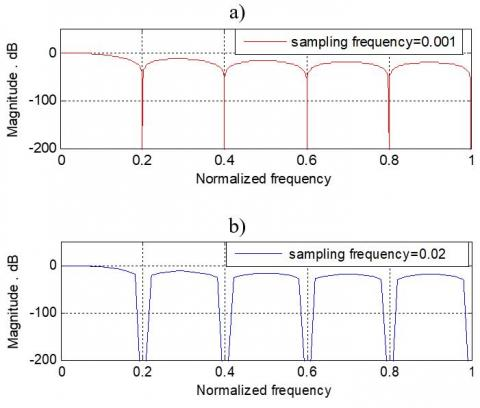Figure 5. CIC filter gain response for two different value of sampling frequency (M=1, N=10)

Now, in order to show the influence of the sampling frequency in the CIC filter characteristics, we show in Figure 5 two gain responses with two different sampling frequency. We notice that a smaller sampling frequency results a faster passband drop.

Based on all aforementioned characteristics of the CIC filter behavior, we are looking for a useful relation between $\Omega$ and N for improving the CIC filter gain response through its parameters. In the next section, we detail the mathematical formulation of objective function in order to find the optimal CIC filter parameters.

3. Proposed Method

The Gain response of the CIC filters depends on three parameters: the decimation factor N, the number of stages M, and the sampling frequency. In this paper, we propose a new method that can resolve the CIC filter problems mentioned-above by optimizing its parameters simultaneously. For this end, we consider the CIC filter Gain response reported in Eq. (3):

$\left|\mathrm{H}_{\mathrm{CIC}}(\Omega, \mathrm{N})\right|=\left|(1 / N) \frac{\sin (\mathrm{N} \Omega / 2)}{\sin \left(\frac{\Omega}{2}\right)}\right|$  (3)

To find the optimal solution for Ω and N, we should compute the partial derivative of Eq. (3) with respect to Ω and N. Straightforward mathematical manipulations allow to write:

$\frac{\partial H(N, \Omega)}{d \Omega}$$=\frac{(N / 2) \cos \left(\frac{\Omega N}{2}\right) \sin \left(\frac{\Omega}{2}\right)-(1 / 2) \cos \left(\frac{\Omega}{2}\right) \sin \left(\frac{\Omega N}{2}\right)}{\sin \left(\frac{\Omega}{2}\right)^{2}} (4) \frac{\partial H(N, \Omega)}{d N}=\frac{(\Omega / 2) \cos \left(\frac{\Omega N}{2}\right) \sin \left(\frac{\Omega}{2}\right)}{\sin \left(\frac{\Omega}{2}\right)^{2}} (5) After adding Eq. (4) to Eq. (5) and setting all to zero, we get: \frac{\left(\frac{N}{2}\right) \cos \left(\frac{\Omega N}{2}\right) \sin \left(\frac{\Omega}{2}\right)-(1 / 2) \cos \left(\frac{\Omega}{2}\right) \sin \left(\frac{\Omega N}{2}\right)}{\sin \left(\frac{\Omega}{2}\right)^{2}}$$+\frac{\left(\frac{\Omega}{2}\right) \cos \left(\frac{\Omega N}{2}\right) \sin \left(\frac{\Omega}{2}\right)}{\sin \left(\frac{\Omega}{2}\right)^{2}}=0$   (6)

After some mathematical manipulations, we obtain:

$\frac{\left(\frac{N}{2}+\frac{\Omega}{2}\right) \cos \left(\frac{\Omega N}{2}\right) \sin \left(\frac{\Omega}{2}\right)-(1 / 2) \cos \left(\frac{\Omega}{2}\right) \sin \left(\frac{\Omega N}{2}\right)}{\sin \left(\frac{\Omega}{2}\right)^{2}}$=0   (7)

We define A= $\left(\frac{\mathrm{N}}{2}+\frac{\Omega}{2}\right)$, hence Eq. (7) becomes:

$\frac{\left(\frac{A}{2}\right)\left[\sin \left(\frac{\Omega}{2}+\frac{\Omega N}{2}\right)+\sin \left(\frac{\Omega}{2}-\frac{\Omega N}{2}\right)\right]-(1 / 2)(1 / 2)\left[\sin \left(\frac{\Omega}{2}+\frac{\Omega N}{2}\right)+\sin \left(\frac{\Omega}{2}-\frac{\Omega N}{2}\right)\right]}{\sin \left(\frac{\Omega}{2}\right)^{2}}=0$  (8)

$\frac{\left(\frac{\mathrm{A}}{2}-\frac{1}{4}\right)\left[\sin \left(\frac{\Omega}{2}+\frac{\Omega \mathrm{N}}{2}\right)\right]+\left(\frac{\mathrm{A}}{2}-\frac{1}{4}\right)\left[\sin \left(\frac{\Omega}{2}-\frac{\Omega \mathrm{N}}{2}\right)\right]}{\sin \left(\frac{\Omega}{2}\right)^{2}}$=0   (9)

$\left(\frac{\mathrm{A}}{2}-\frac{1}{4}\right)\left[\sin \left(\frac{\Omega}{2}+\frac{\Omega \mathrm{N}}{2}\right)\right]$$+\left(\frac{\mathrm{A}}{2}-\frac{1}{4}\right)\left[\sin \left(\frac{\Omega}{2}-\frac{\Omega \mathrm{N}}{2}\right)\right]=0 (10) \sin \left(\frac{\Omega}{2}+\frac{\Omega N}{2}\right)+\sin \left(\frac{\Omega}{2}-\frac{\Omega N}{2}\right)=0 (11) \sin \left(\frac{\Omega}{2}+\frac{\Omega N}{2}\right)=-\sin \left(\frac{\Omega}{2}-\frac{\Omega N}{2}\right) (12) Now, we involve the following trigonometric rule to resolve Eq. (12): \sin (\pi+\theta)=-\sin (\theta)=\sin ((2 k+1) \pi+\theta) (13) where, k is a positive integer. Accordingly, we can write: \sin \left(\frac{\Omega}{2}+\frac{\Omega \mathrm{N}}{2}\right)=\sin \left(\left(\frac{\Omega}{2}-\frac{\Omega \mathrm{N}}{2}\right)+(2 \mathrm{k}+1) \pi\right) (14) \left(\frac{\Omega}{2}+\frac{\Omega N}{2}\right)=\left(\left(\frac{\Omega}{2}-\frac{\Omega N}{2}\right)+(2 k+1) \pi\right) (15) Finally, the optimal value of Ω in function of N is as follows: \Omega=(2 k+1) \pi / N (16) 4. Results and Discussion In this section, we carry out our experiments on the proposed CIC filter gain response using Matlab software. We perform several values of Ω as function of N according to Eq. (16) to compute the gain response expressed in Eq. (2). In order to demonstrate the effectiveness of the proposed method, we opted for the following tests: Test 1: In this example, we designed a CIC filter gain response for M=1 and four different values of N (48, 64, 128, and 256). Figure 5 shows the magnitude responses of the proposed filter in function of N. We Note that there is a significant improvement in both stopband attenuation and ripple while increasing the value of N. Test 2: Now, we increase the number of stages from 1 to 2 while keeping the same values of N as in test 1. Then, we plot the obtained gain response in Figure 7, which seems the same one shown in the first test (Figure 6) but with the minimum stopband attenuation doubling. Test 3: For three stages in CIC filter (As shown in Figure 8), we notice that the passband of the gain responses with the four values of N are rapidly dropped compared to ones performed in test 1 and 2. Test 4: As shown in Figure 9, we take M=4 while keeping the same values of N as in the previous tests. We clearly notice that the passband droop is faster than ones obtained before. This behavior can significantly improve the amplitude response of the CIC filter with rational number of stages M (a cost constraint). a) ## 6a.jpg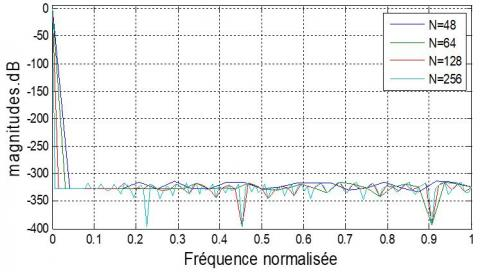b) ## 6b.jpg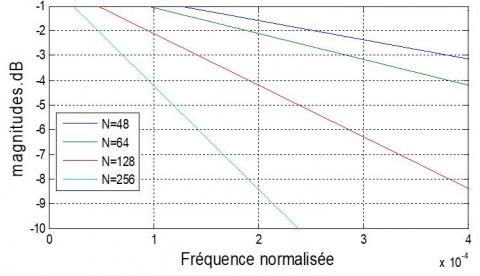Figure 6. Gain response of the proposed CIC filter with single stage (M=1) and four values of N: a) the whole magnitude response, b) the passband zoom a) ## 7a.jpg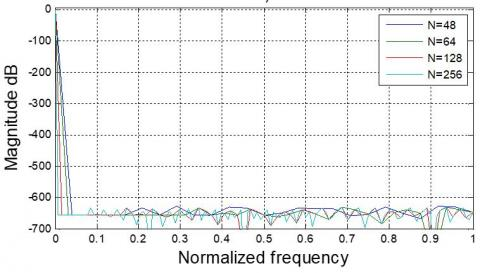b) ## 7b.jpg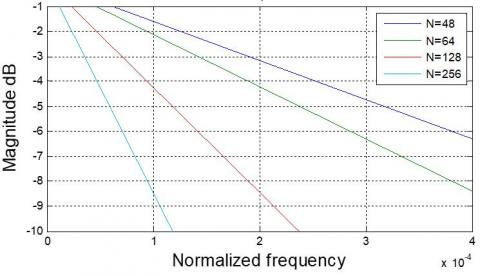Figure 7. Gain response of the proposed CIC filter with two stages (M=2) and four values of N: a) the whole magnitude response, b) the passband zoom a) ## 8a.jpg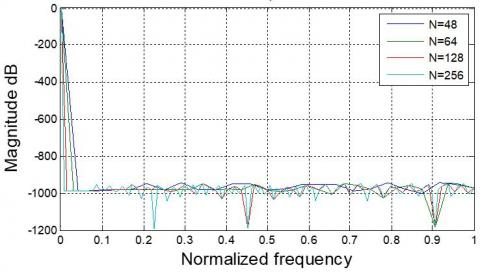b) ## 8b.jpg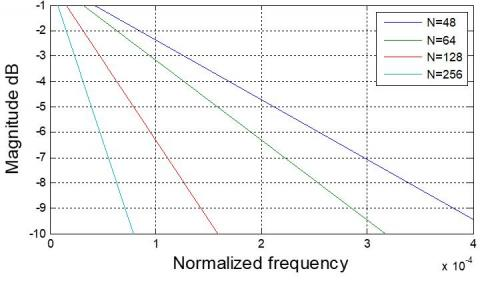Figure 8. Gain response of the proposed CIC filter with three stages (M=3) and four values of N: a) the whole magnitude response, b) the passband zoom a) ## 9a.jpg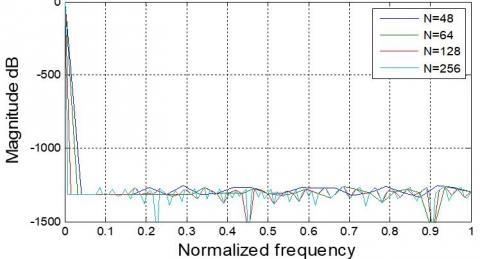b) ## 9b.jpg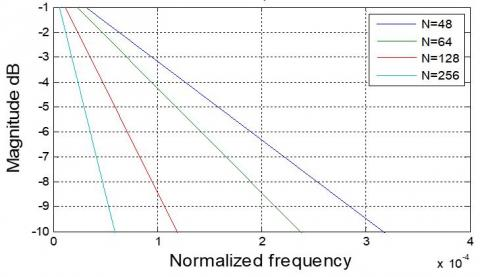Figure 9. Gain response of the proposed CIC filter with four stages (M=4) and four values of N: a) the whole magnitude response, b) the passband zoom 5. Comparison In order to make a meaningful performance assessment of the proposed method compared to other existing CIC filters, we compare the gain response of our proposed method with three other existing CIC filters namely: the conventional CIC filter expressed in Eq. (2), the sharpened CIC filter , and the modified sharpened CIC filter . The frequency responses of the sharpened (H_{s h}) and the modified sharpened (H_{M s h}) CIC filters, with which we compare, are given in the following equations: \left|H_{s h}(\Omega, \mathrm{N}, \mathrm{M})\right|=\left|3\left[\frac{1}{N} \frac{\sin (\mathrm{N} \Omega / 2)}{\sin \left(\frac{\Omega}{2}\right)}\right]^{2 M}-2\left[\frac{1}{N} \frac{\sin (\mathrm{N} \Omega / 2)}{\sin \left(\frac{\Omega}{2}\right)}\right]^{3 M}\right| (17) \left|H_{M s h}\left(\Omega, N, N_{1}, N_{2}, M, L\right)\right|$$=\mid\left[\frac{1}{N_{1}} \frac{\sin \left(N_{1} \Omega / 2\right)}{\sin \left(\frac{\Omega}{2}\right)}\right]^{L}\left\{3\left[\frac{1}{N_{2}} \frac{\sin (N \Omega / 2)}{\sin \left(N_{1} \frac{\Omega}{2}\right)}\right]^{2 M}\right.$$\left.-2\left[\frac{1}{N_{2}} \frac{\sin (N \Omega / 2)}{\sin \left(N_{1} \frac{\Omega}{2}\right)}\right]^{3 M}\right) \mid$   (18)

where, $\mathrm{N}_{1}$ is the decimation factor of the comb filter section, $\mathrm{N}_{2}$ is the decimation factor of the sharpened filter section so that $\mathrm{N}=\mathrm{N}_{1} * \mathrm{~N}_{2}$, and $\mathrm{L} \geq 2 \mathrm{M}$. We note that $\mathrm{N}_{1}, \mathrm{~N}_{2}, \mathrm{M}, \mathrm{L}$ are integers. For more details about sharpened and modified sharpened CIC filter structures, the interested reader should consult these references [12-14].

## 10.jpg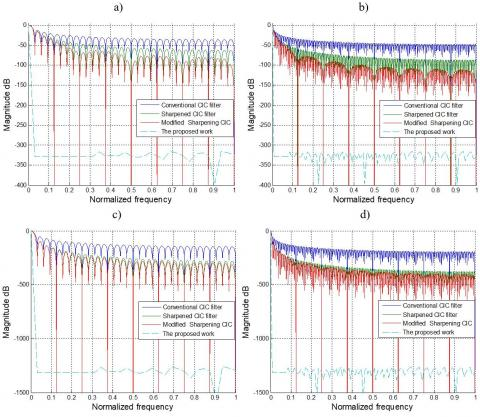Figure 10. Frequency response comparison with the following parameters: a) M=1, N=64, N1=N2=8, L=3, b) M=1, N=256, N1=N2=16, L=3, c) M=4, N=64, N1=N2=8, L=9, and d) M=4, N=256, N1=N2=16, L=9

Table 1. Comparison of stopband attenuation

 Filter type Parameters Stopband attenuation (dB) M N N1 N2 L Conventional CIC  1 64 - - - 13.26- 128 - - - -13.27 256 - - - 13.28- 2 64 - - - -26.52 128 - - - -26.56 256 - - - -26.55 3 64 - - - -39.77 128 - - - -39.84 256 - - - -39.85 4 64 - - - 53.03- 128 - - - -53.13 256 - - - 53.13- Sharpened CIC  1 64 - - - 15.8- 128 - - - 15.8-3 256 - - - 15.85- 2 64 - - - -43.77 128 - - - -43.86 256 - - - -43.86 3 64 - - - -69.95 128 - - - -70.09 256 - - - -70.09 4 64 - - - 96.54- 128 - - - -96.72 256 - - - 96.73- Modified Sharpened CIC  1 64 8 8 3 16.2- 128 16 8 3 -16.24 256 16 16 3 15.95- 2 64 8 8 5 -44.26 128 16 8 5 -44.33 256 16 16 5 -43.98 3 64 8 8 7 -70.4 128 16 8 7 -70.52 256 16 16 7 -70.2 4 64 8 8 9 97.01- 128 16 8 9 -97.17 256 16 16 9 96.84- The proposed method 1 64 - - - 315- 128 - - - 315-.4 256 - - - 315.2- 2 64 - - - -630.4 128 - - - -630.4 256 - - - -630.4 3 64 - - - -945.6 128 - - - -946.3 256 - - - -945.6 4 64 - - - 1261- 128 - - - -1261 256 - - - 1261-

From Figure 10, we clearly notice the effectiveness of the proposed method in terms of stopband attenuation and ripple whatever the values of N and M. Furthermore, the passband droop is considerably improved compared to others. This superiority can be justified by the optimizing of the CIC filter parameters so that this solution examined the effect of sampling rate on the CIC filter gain response and found its optimal value which improved efficiently the CIC filter magnitude response.

Table 1 summarizes the performance of the proposed method compared to the other existing CIC filters in term of stopband attenuation statistics. It is clear that the sharpened and the modified sharpened CIC filter performances are almost similar but the both are better than conventional CIC filter. However, the proposed method gives the best stopband attenuation which is 13 times better than that of modified sharpened CIC filter. These statistics make our optimized CIC filter the best choice for improving the characteristics of the decimation filtering.

6. Conclusions

We have presented in this paper a new method for improving the CIC filter characteristics. This opted solution has examined the effect of sampling rate on the CIC filter gain response by finding the optimal sampling rate value which improve efficiently the CIC filter characteristics. The evaluated results of the proposed CIC filter confirmed the enhancements in both stopband attenuation and ripple as well as the passband droop. Besides, the comparison carried out between our method and some other existing CIC filters showed the effect of the sampling rate parameter on both stopband and passband performances. This improvement makes our CIC filter suitable for many signal processing applications, especially in wireless domain including WCDMA and WiMAX with different parameter combinations.

For future work, we are planning to investigate more deeply various sampling rate-based optimization method such as Lagrangian constrained optimization for improving the CIC filter characteristics.

References

 Chergui, L., Bouguezel, S. (2019). A new post-whitening transform domain LMS algorithm. Traitement du Signal, 36(3): 245-252. https://doi.org/10.18280/ts.360307

 De Matteis, M., Pezzotta, A., D'Amico, S., Baschirotto, A. (2015). A 33 MHz 70 dB-SNR super-source-follower-based low-pass analog filter. IEEE Journal of Solid-State Circuits, 50(7): 1516-1524. https://doi.org/10.1109/JSSC.2015.2411626

 Yuce, E., Minaei, S. (2009). ICCII-based universal current-mode analog filter employing only grounded passive components. Analog Integrated Circuits and Signal Processing, 58(2): 161-169. https://doi.org/10.1007/s10470-008-9225-2

 Hogenauer, E. (1981). An economical class of digital filters for decimation and interpolation. IEEE Transactions on Acoustics, Speech, and Signal Processing, 29(2): 155-162. https://doi.org/10.1109/TASSP.1981.1163535

 Sengar, I.S., Bhattacharya, P.P. (2012). Performance evaluation of cascaded integrator-comb (CIC) filter. IOSR Journal of Engineering (IOSRJEN), 2(2): 222-228. https://doi.org/10.9790/3021-0202222228

 Saravanan, P.D., Jayaprakasan, V. (2013). Design and implementation of efficient CIC filter structure for decimation. International Journal of Computer Applications, 65(14): 1-7.

 Jayaprakasan, V., Madheswaran, M. (2013). Cascading sharpened CIC and polyphase FIR filter for decimation filter. In Proceedings2nd International Conference on Advances in Electrical and Electronics Engineering ICAEE, pp. 148-154.

 Mishra, V.K., Baig, M.A. (2015). Design of sixth order CIC compensation filter. 2015 IEEE Power, Communication and Information Technology Conference (PCITC), Bhubaneswar, India, pp. 535-538. https://doi.org/10.1109/PCITC.2015.7438223

 Sinha, D., Kumar, S. (2016). CIC filter for sample rate conversion in software defined radio. 2016 World Conference on Futuristic Trends in Research and Innovation for Social Welfare (Startup Conclave), Coimbatore, India, pp. 1-5. https://doi.org/10.1109/STARTUP.2016.7583972

 Chukwuchekwa, N., Akinwole, B., Onojo, J. (2018). Enhancement of the performance characteristics of CIC decimation filters for multirate DSP applications. IOSR Journal of VLSI and Signal Processing (IOSR-JVSP) 8(6-I): 01-09. https://doi.org/10.9790/4200-0806010109

 Kwentus, A.Y., Jiang, Z., Willson, A.N. (1997). Application of filter sharpening to cascaded integrator-comb decimation filters. IEEE Transactions on Signal Processing, 45(2): 457-467. https://doi.org/10.1109/78.554309

 Jeong, C., Min, Y.J., Kim, S.W. (2011). Double‐sharpened decimation filter employing a pre‐droop compensator for multistandard wireless applications. ETRI Journal, 33(2): 169-175. https://doi.org/10.4218/etrij.11.0110.0272

 Molnar, G., Dudarin, A., Vucic, M. (2017). Design and multiplierless realization of maximally flat sharpened-CIC compensators. IEEE Transactions on Circuits and Systems II: Express Briefs, 65(1): 51-55. https://doi.org/10.1109/TCSII.2017.2700081